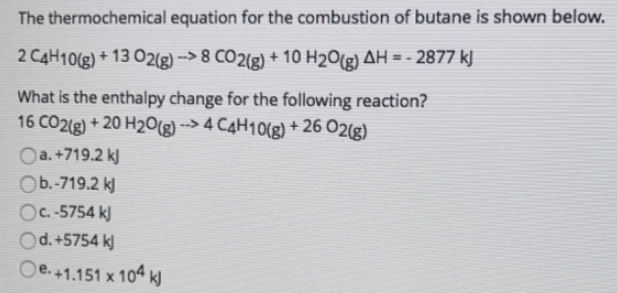# Problem: The thermochemical equation for the combustion of butane is shown below. 2C4H10 (g) + 13O2 (g) → 8CO2 (g) + 10H2O (g)     ΔH = -2877 k What is the enthalpy change for the following reaction? 16CO2 (g) + 20H2O (g) → 4C4H10 (g) + 26O2 (g) a. +719.2 kjb. -719.2 kj c. -5754 kj d. +5754 kj e. +1.151 x 104 kj

🤓 Based on our data, we think this question is relevant for Professor Gold's class at UTSA.

###### FREE Expert Solution###### Problem Details

The thermochemical equation for the combustion of butane is shown below.

2C4H10 (g) + 13O2 (g) → 8CO2 (g) + 10H2O (g)     ΔH = -2877 k

What is the enthalpy change for the following reaction?

16CO2 (g) + 20H2O (g) → 4C4H10 (g) + 26O2 (g)

a. +719.2 kj

b. -719.2 kj

c. -5754 kj

d. +5754 kj

e. +1.151 x 104 kj